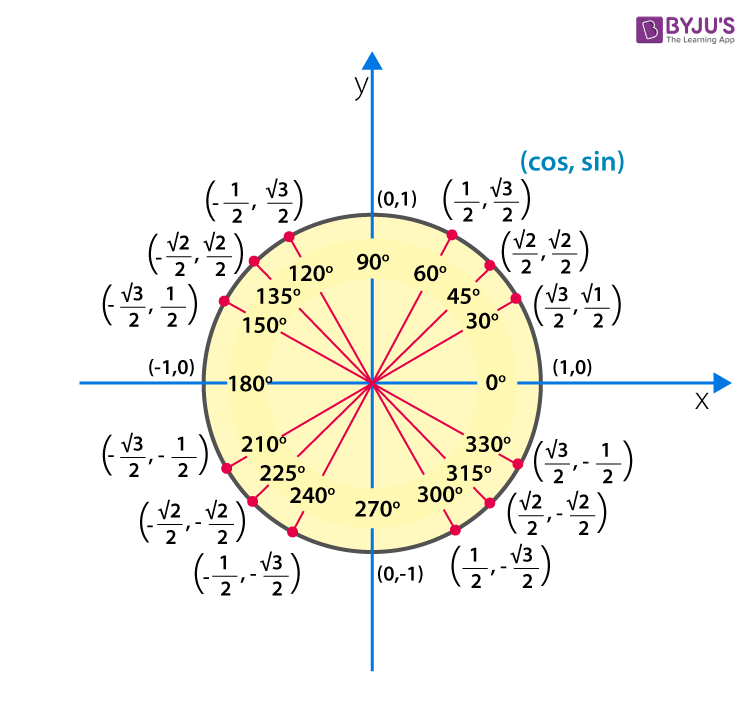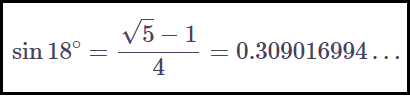# Sin 18

In trigonometry, the sine is one of the six important trigonometric ratios. This can be denoted as “sin” while writing the values for different angles. We can find the value of sine for several degree measures such as 0°, 30°, 45°, 60°, 90°, etc.,

The below figure shows the value of sine for different measures of angles, such as sin 180 degrees.The above figure helps in finding the other degree values for the sine and also helps in solving many trigonometry problems. In this article, you will learn how to find the value of sin 18 degrees and sin 18 radians with detailed explanations.

## Sin 18 Degrees

The value of the sine function at an angle of 180 degrees is called Sin 18 degrees and it can be written as “sin 18°”. In a circular system, sin 18 degrees can be written as sin π/10. The value of sin 18° is given by:Let’s understand how to calculate the value of sin 18 degrees here.

## How to Find Sin 18 Degree Value

Let θ = 18°….(i)

We can write 90° as:

90° = 36° + 54°

90 = 2 × 18° + 3 × 18°

Substituting (i) in the above equation,

90° = 2θ + 3θ

3θ = 90° – 2θ

Taking “sin” on both sides,

sin 3θ = sin(90° – 2θ)

Using the identity sin 3A = 3 sin A – 4 sin3A,

3 sin θ – 4 sin3θ = cos 2θ

Now, using the identity cos 2θ = 1 – 2 sin2θ,

3 sin θ – 4 sin3θ = 1 – 2 sin2θ = 0

4 sin3θ – 2 sin2θ – 3 sin θ + 1 = 0

Let us assume sin θ = x.

Thus,

4x3 – 2x2 – 3x + 1 = 0

Using factor method, we can write the above equation as:

(x – 1)(4x2 + 2x – 1) = 0

x – 1 = 0, 4x2 + 2x – 1 = 0

x = 1, 4x2 + 2x – 1 = 0

We know that -1 ≤ sin ≤ 1, where sine is maximum at 90 degrees.

Thus, sin 18 value will be less than 1.

So, x = 1 cannot be accepted.

Now,

4x2 + 2x – 1 = 0

x = [-2 ± √{4 – 4(-4)}]/2(4)

= [-2 ± √(4 + 16)]/8

= [-2 ± √20]/8

= [-2 ± 2√5]/8

= 2[-1 ± √5]/8

= (-1 ± √5)/4

The value of sin θ is positive when θ lies between 0 and 90 degrees.

Therefore, x = sin θ = sin 18° = (-1 + √5)/4

Or

sin 18° = (√5 – 1)/4

### Sin 18 Degrees in Fraction

The value of sin 18 degrees can be expressed in terms of a fraction as given below:

sin 18° = (√5 – 1)/4

= (2.23606798… – 1)/4

= 1.23606798…/4

= 0.309016995…

Thus, sin 18° = 0.309016995…

If we consider the approximate (rounded to 3 digits) of the √5, then;

sin 18 = (2.236 – 1)/4

= 1.236/4

= 0.309

Therefore, sin 18° = 0.31 or 31/100

### Sin 18 Value

Sin 18 value in radians is equal to -0.769214 (approx). This value can be calculated by converting the radians measure value to degree measure.

### How to Find Sin 18 Value

We know that,

That means,

Thus, sin 18 rad = sin 1031.32°

Using trigonometric functions calculator, we can get the value of sin 1031.32°.

Hence, sin 18 rad = 0.769214…

We know that 1031.32° lies in the fourth quadrant, where sin is negative.

Therefore, sin 18 rad = -0.769214…

Also, we can represent the value of sin 18 in fraction in the same way as we did in the case of sin 18 degrees.

### Cos 18 Value

Now, let’s learn how to find the value of cos 18 degrees.

Consider the identity sin2A + cos2A = 1,

Let us take A = 18°.

sin2(18°) + cos2(18°) = 1

cos2(18°) = 1 – sin2(18°)

= 1 – [(√5 – 1)/4]2

= 1 – [(1 + 5 – 2√5)/16]

= [16 – 6 + 2√5]/16

= (10 + 2√5)/16

Therefore,

$cos 18^{\circ}=\sqrt{\frac{10+2\sqrt{5}}{16}}\\=\frac{\sqrt{10+2\sqrt{5}}}{4}$

Using the sin of 18 degrees value, we can write the other sin values as given in the below table.

 18° 36° 54° 72° sin $\frac{\sqrt{5}-1}{4}$ $\frac{\sqrt{10-2\sqrt{5}}}{4}$ $\frac{\sqrt{5}+1}{4}$ $\frac{\sqrt{10+2\sqrt{5}}}{4}$ cos $\frac{\sqrt{10+2\sqrt{5}}}{4}$ $\frac{\sqrt{5}+1}{4}$ $\frac{\sqrt{10-2\sqrt{5}}}{4}$ $\frac{\sqrt{5}-1}{4}$ tan $\frac{\sqrt{5}-1}{\sqrt{10+2\sqrt{5}}}$ $\frac{\sqrt{10-2\sqrt{5}}}{\sqrt{5}+1}$ $\frac{\sqrt{5}+1}{\sqrt{10-2\sqrt{5}}}$ $\frac{\sqrt{10+2\sqrt{5}}}{\sqrt{5}-1}$

These values help in solving different trigonometry problems in maths.

### Sin 18 Degree by Cos 72 Degree

Let’s calculate the value of sin 18 upon cos 72 degrees.

sin 18°/cos 72°

= [(√5 – 1)/4]/ [(√5 – 1)/4]

= 1

Alternatively,

sin 18°/cos 72° = sin 18°/cos(90° – 18°)

= sin 18°/sin 18°

= 1

Therefore, sin 18° by cos 72° is equal to 1.

### Sin 18 Cos 36

sin 18° cos 36° = [(√5 – 1)/4] [(√5 + 1)/4] [(√5)2 – 12]/16

= (5 – 1)/16

= 4/16

= 1/4

Therefore, sin 18° cos 36° is equal to 1/4.

### Sin 18 Sin 54

sin 18° sin 54° = [(√5 – 1)/4] [(√5 + 1)/4] [(√5)2 – 12]/16

= (5 – 1)/16

= 4/16

= 1/4

Therefore, sin 18° sin 54° is equal to 1/4.

To get the values of sin for other angle measures, visit www.byjus.com and practice a number of questions on trigonometry.